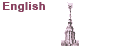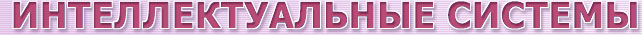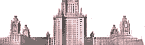Официальный сайт кафедры Математической теории интеллектуальных систем и лабораторий Проблем теоретической кибернетики и Математичеких проблем искусственного интеллекта механико-математического факультета МГУ им. М. В. Ломоносова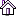Новости Кафедра Сотрудники Учеба Наука Исследования Журнал Культура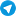# On one method to decrease average search time

## Elyar E. Gasanov and Irina V. KuznetsovaMoscow State University, Russia

We will consider two-dimensional range searching. Let Y=[0,1]x[0,1]. X={x=(u,v,w,z): 0<u<v<1, 0<w<z<1} be a set of inquires. r – relation on XxY such that for any x=(u,v,w,z) from X and y=(p,q) from Y x r y if and only if u<p<v & w<q<z. V be a finite subset of Y. Two-dimensional range searching consists in enumeration for any inquire x from X all that and only that objects y from V, such that x r y. Three characteristics (amount of memory, worst-case search time and average search time) are usually used for estimation of search algorithms. Say search algorithm is (f(k),g(k),h(k))-algorithm if amount of memory, worst-case search time and average search time of this algorithm are equal to O(f(k)), O(g(k)) and O(h(k)) correspondingly when k becomes infinite, there k=|V|. (exp(3 ln k), log k, log k)-algorithm  and (exp((1+a) ln k), log k, log k)-algorithm (0<a<1) ,solving two-dimensional range searching, are known. We suggest some method of modification of these algorithms, which leads to (exp(3 ln k), log k, 1)-algorithm  and (exp((1+a) ln k), log k, 1)-algorithm.

Reference:

 J.L.Bentley, Decomposable searching problems. Info. Proc. Lett. (1979) 8, 244-251.

 J.L.Bentley, H.A.Mayrer, Efficient worst-case data structures for range searching. Acta

Informatica (1980) 13, 155-168.

 E.E.Gasanov, Instantly solvable search problems. Discrete Mathematics and Applications

(1996) 6, 467-482.

Abstracts of I Turkish World Mathematics Symposium Elazig, Turkey (29 June – 2 July 1999).

Наверх© 2001- г. Кафедра Математической теории интеллектуальных систем, лаборатория ПТК, лаборатория МПИИ Написать вебмастеруПоследние новости - в телеграм-канале кафедры МаТИС: